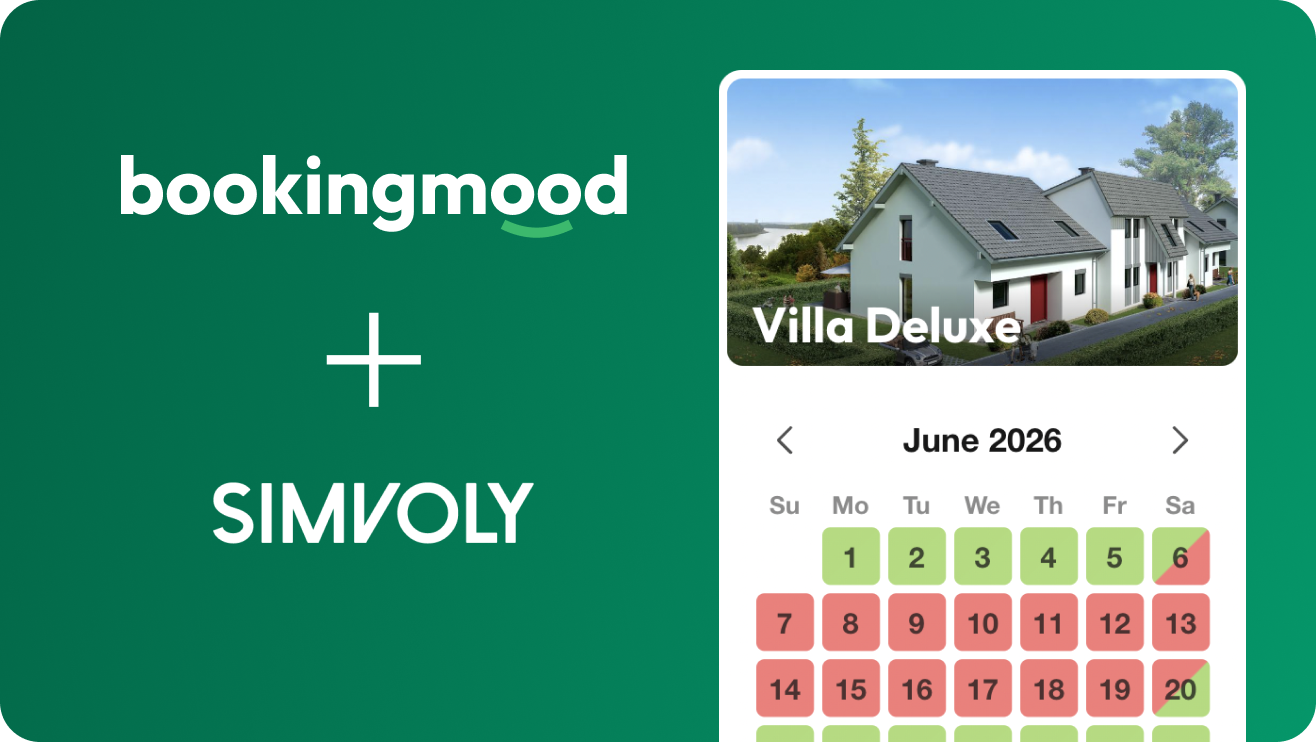# Booking calendar for Simvoly## Looking for a booking calendar for Simvoly?self.__wrap_n=self.__wrap_n||(self.CSS&&CSS.supports("text-wrap","balance")?1:2);self.__wrap_b=(e,t,n)=>{let r=(n=n||document.querySelector(`[data-br="\${e}"]`)).parentElement,i=e=>n.style.maxWidth=e+"px";n.style.maxWidth="";let o=r.clientWidth,a=r.clientHeight,l=o/2-.25,s=o+.5,c;if(o){for(i(l),l=Math.max(n.scrollWidth,l);l+1<s;)i(c=Math.round((l+s)/2)),r.clientHeight===a?s=c:l=c;i(s*t+o*(1-t))}n.__wrap_o||"undefined"!=typeof ResizeObserver&&(n.__wrap_o=new ResizeObserver(()=>{self.__wrap_b(0,+n.dataset.brr,n)})).observe(r)};self.__wrap_n!=1&&self.__wrap_b(":R5aqpamlla:",1)

Bookingmood is a simple, flexible booking calendar that can be added to your Simvoly website in just a few mouse clicks. No technical skills are required; it all works out of the box. We wrote a simple step-by-step manual for Simvoly as well.

Check the manual

### About bookingmoodself.__wrap_n=self.__wrap_n||(self.CSS&&CSS.supports("text-wrap","balance")?1:2);self.__wrap_b=(e,t,n)=>{let r=(n=n||document.querySelector(`[data-br="\${e}"]`)).parentElement,i=e=>n.style.maxWidth=e+"px";n.style.maxWidth="";let o=r.clientWidth,a=r.clientHeight,l=o/2-.25,s=o+.5,c;if(o){for(i(l),l=Math.max(n.scrollWidth,l);l+1<s;)i(c=Math.round((l+s)/2)),r.clientHeight===a?s=c:l=c;i(s*t+o*(1-t))}n.__wrap_o||"undefined"!=typeof ResizeObserver&&(n.__wrap_o=new ResizeObserver(()=>{self.__wrap_b(0,+n.dataset.brr,n)})).observe(r)};self.__wrap_n!=1&&self.__wrap_b(":Rbaqpamlla:",1)

Do you want to accept bookings or just display the availability? Bookingmood can do both, but it's your choice. That's what we call flexibility! From a simple availability calendar to an automated booking system: Bookingmood has a right plan for you as a Simvoly user.